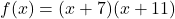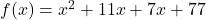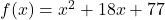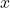## what is the shape and direction of f(x)=(x+7)(x+11)

Question

what is the shape and direction of f(x)=(x+7)(x+11)

in progress 0
6 months 2021-07-30T12:11:58+00:00 1 Answers 5 views 0

1. Given:

The function isTo find:

The shape and direction of the given function.

Solution:

We have,It can be written asIt is a quadratic function and the power ofis 2 so the graph of this function is a vertical parabola.

The leading coefficient is positive so the parabola opens upward.

Therefore, the given function is a vertical parabola that opens upward.